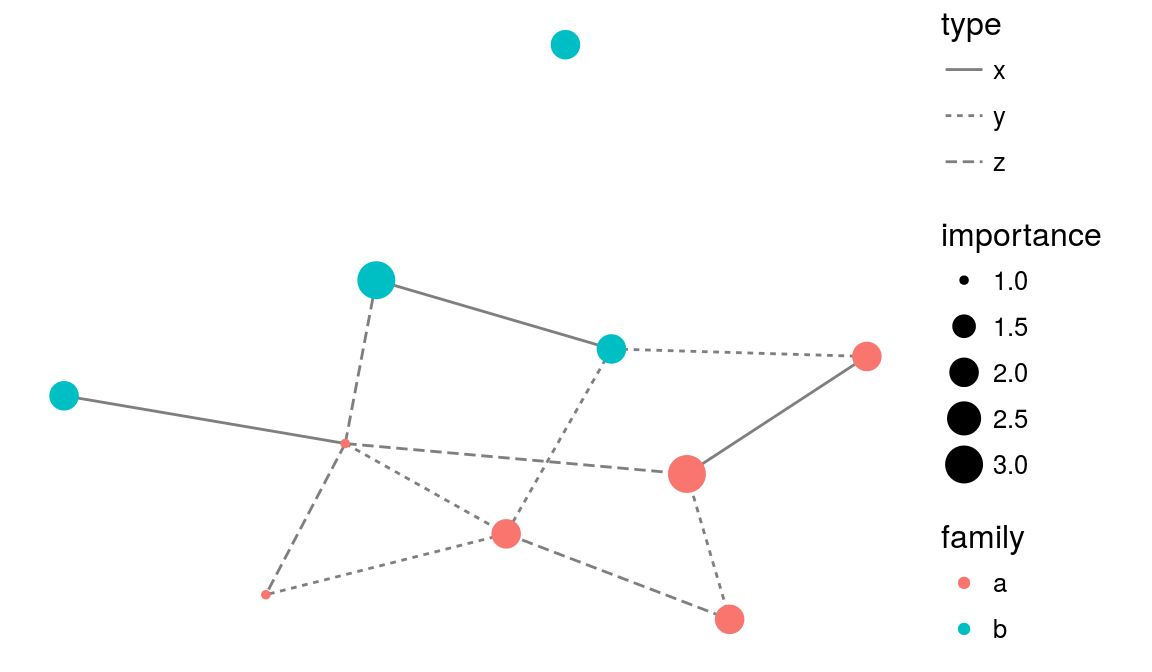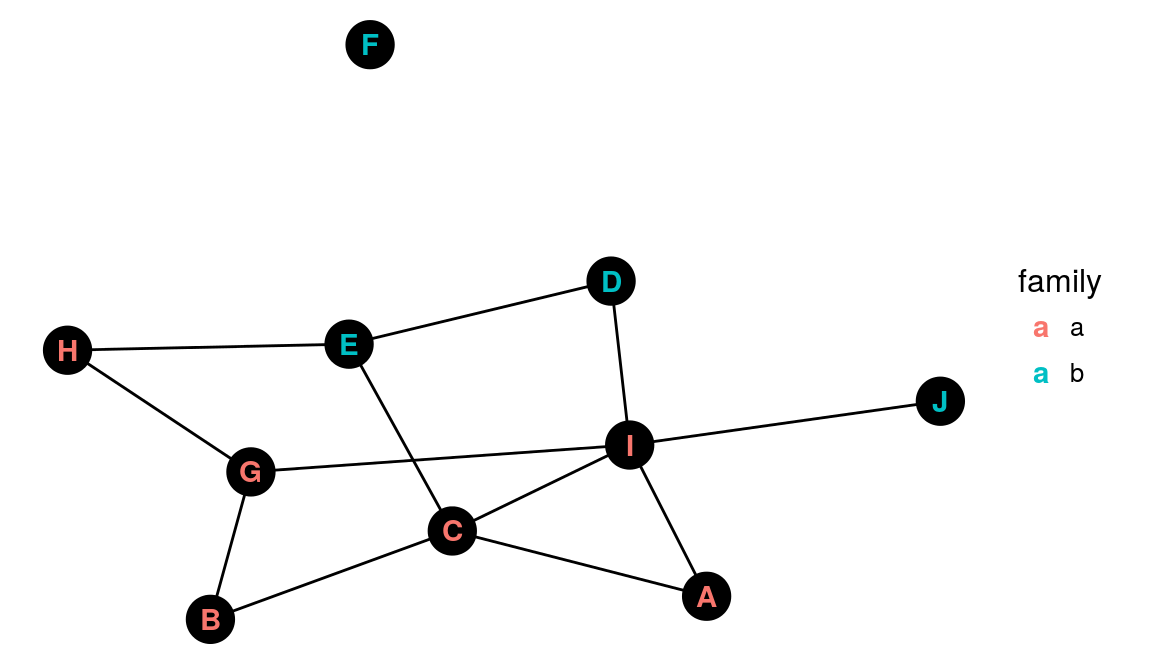ggplot2 now has an official extension mechanism. This means that others can now easily create their own stats, geoms and positions, and provide them in other packages. This should allow the ggplot2 community to flourish, even as less development work happens in ggplot2 itself. This page showcases these extensions.

### ggnetwork

https://github.com/briatte/ggnetwork

The ggnetwork package provides a way to build network plots with ggplot2.

``````# Example from https://briatte.github.io/ggnetwork/
library(ggplot2)
library(ggnetwork)

# Let’s define a small random graph to illustrate each component of ggnetwork:
library(network)
library(sna)
n = network(rgraph(10, tprob = 0.2), directed = FALSE)

# Let’s now add categorical and continuous attributes for both edges and vertices
n %v% "family" = sample(letters[1:3], 10, replace = TRUE)
n %v% "importance" = sample(1:3, 10, replace = TRUE)

# We now add a categorical edge attribute called "type",
# which is set to either "x", "y" or "z", and a continuous vertex
# attribute called "day", which is set to either 1, 2 or 3.
e = network.edgecount(n)
set.edge.attribute(n, "type", sample(letters[24:26], e, replace = TRUE))
set.edge.attribute(n, "day", sample(1:3, e, replace = TRUE))

# Let’s now draw the network edges using geom_edges
# theme_blank
ggplot(data = ggnetwork(n), aes(x = x, y = y, xend = xend, yend = yend)) +
geom_edges(aes(linetype = type), color = "grey50") +
theme_blank()````````````# Let’s now draw the nodes using geom_nodes
ggplot(data = ggnetwork(n), aes(x = x, y = y, xend = xend, yend = yend)) +
geom_edges(aes(linetype = type), color = "grey50") +
geom_nodes(aes(color = family, size = importance)) +
theme_blank()````````````# Let’s now add node labels.
ggplot(data = ggnetwork(n), aes(x = x, y = y, xend = xend, yend = yend)) +
geom_edges(color = "black") +
geom_nodes(color = "black", size = 8) +
geom_nodetext(aes(color = family, label = LETTERS[ vertex.names ]), fontface = "bold") +
theme_blank()``````